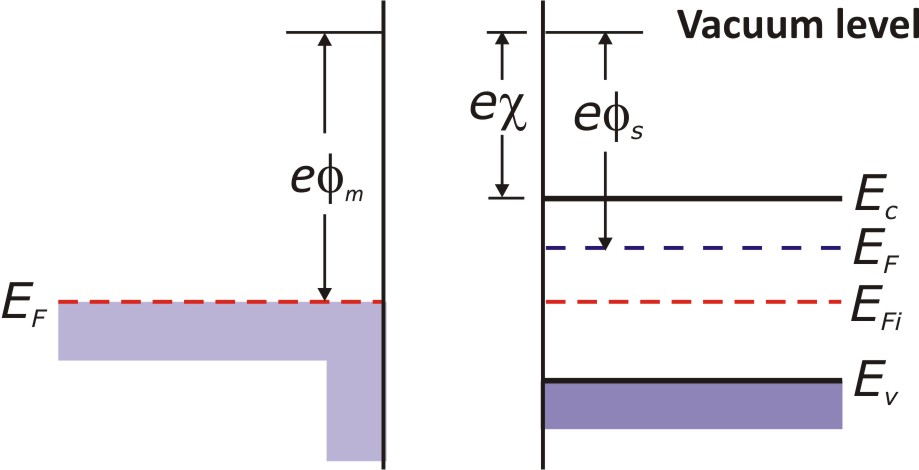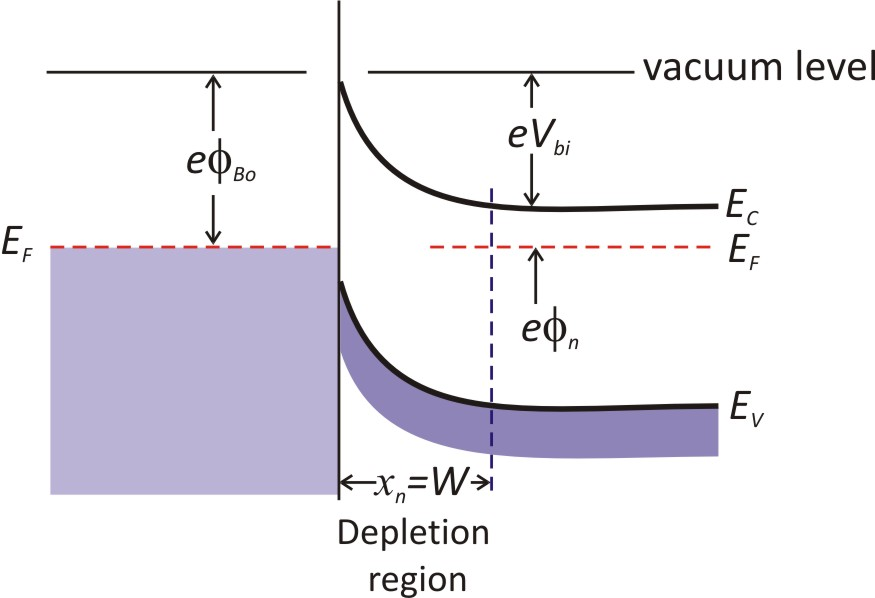# Schottky Contact

The rectifying contact that occur between a metal and a lightly doped semiconductor.a)                                                                      b)

Figure(1) a) Energy band diagram of a metal and semiconductor before contact,
 b) Ideal energy -band diagram of a metal and n-type semiconductor junction.

If a metal work function$\phi_m$ is larger than a semiconductor electron affinity$\chi$, therefore contacting metal to the semiconductor leads to electron flow from the semiconductor to the metal as a prerequisite of Fermi levels alignment and thermal equilibrium. The remaining positively charged atoms in the semiconductor part create a space charge region. Thus electron flows from semiconductor to metal see a barrier of height$\phi_{BO}$ known as Schottky barrier.$\phi_{BO}=(\phi_m-\chi)$